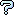All about flooble | fun stuff | Get a free chatterbox | Free JavaScript | Avatarsperplexus dot infoQuadruplets with Square Triplets (Posted on 2005-02-23)Find four distinct positive integers such that the sum of any three of them is a perfect square.

 See The Solution Submitted by David Shin Rating: 4.0000 (3 votes)Comments: ( Back to comment list | You must be logged in to post comments.)re: Number of Solutions (spoilers present)| Comment 7 of 9 |(In reply to Number of Solutions by Robert)

I don't understand what the number of solutions per given square mean, or how they relate to the formulas given.

For example, the line 12^2 maximum indicates 2 solutions. Does this mean {144,  88,  57,  24} is one of them? And how do the formulae given help find the other, and know that only 2 solutions exist? ...and why limit the integers themselves to being perfect squares?  ... and how do the solutions for a given square include those for the previous square?

What would seem easier, and more representative of the formulae given, would be to go in with the maximum square represented.  In that case, no solution has 12^2 as its maximum square represented. The one that has 11^2, for example, as its maximum square represented is {58,  41,  22,  1}.

A program that uses the formulas that Robert provides is

DEFDBL A-Z
DO
INPUT b4
s4 = b4 * b4
FOR b1 = 0 TO b4 - 3
s1 = b1 * b1
FOR b2 = b1 + 1 TO b4 - 2
s2 = b2 * b2
FOR b3 = b2 + 1 TO b4 - 1
s3 = b3 * b3
i1 = (s2 + s3 + s4 - 2 * s1) / 3
i2 = (s1 + s3 + s4 - 2 * s2) / 3
i3 = (s1 + s2 + s4 - 2 * s3) / 3
i4 = (s1 + s2 + s3 - 2 * s4) / 3
IF i1 = INT(i1) AND i2 = INT(i2) AND i3 = INT(i3) AND i4 = INT(i4) THEN
IF i1 > 0 AND i2 > 0 AND i3 > 0 AND i4 > 0 THEN
PRINT i1; i2; i3; i4
END IF
END IF
NEXT
NEXT
NEXT
LOOP

but, again, it finds all sets of numbers whose subtotals add to a maximum of the square of a given number, not ones whose members include a certain square number as their maximum.  Some results are

? 11
58  41  22  1
? 12
? 13
78  57  34  9
? 14
103  59  34  7
89  66  41  14
? 15
130  61  34  5
116  68  41  12
? 16
159  63  34  3
136  88  32  1
145  70  41  10
113  86  57  26
? 17
190  65  34  1
176  72  41  8
151  99  39  6
124  97  68  4
144  88  57  24
126  97  66  33
? 18
209  74  41  6
157  130  37  2
177  90  57  22
159  99  66  31
? 19
244  76  41  4
201  114  46  9
212  92  57  20
183  123  55  18
194  101  66  29
152  121  88  16
154  121  86  49
? 20
281  78  41  2
249  94  57  18
207  114  79  3
231  103  66  27
189  158  53  14
200  136  64  25
167  134  99  23
191  123  86  47
169  134  97  58

The number sets below each are all that are found with the given formulae with their largest subtotal of three being the square of the number above them after the ? prompt.

 Posted by Charlie on 2005-02-24 13:39:08Please log in:

 Search: Search body:
Forums (0)